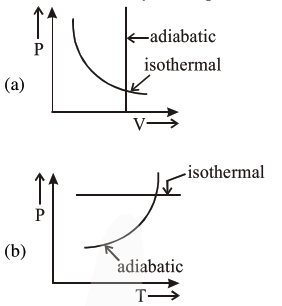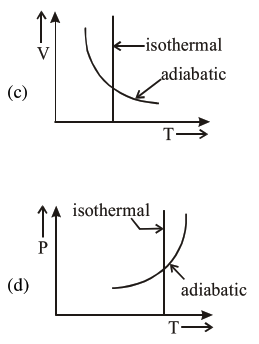# Which one is the correct option`
Question:

Which one is the correct option for the two different thermodynamic processes ?1. (c) and (a)

2. (c) and (d)

3. (a) only

4. (b) and (c)

Correct Option: , 2

Solution:

(2) Option (a) is wrong ; since in adiabatic process $\mathrm{V} \neq$ constant.

Option (b) is wrong, since in isothermal process

$\mathrm{T}=$ constant

Option (c) \& (d) matches isothermes \& adiabatic formula :

$\mathrm{TV}{ }^{\gamma-1}=$ constant $\& \frac{\mathrm{T}^{\gamma}}{\mathrm{p}^{\gamma-1}}=$ constant